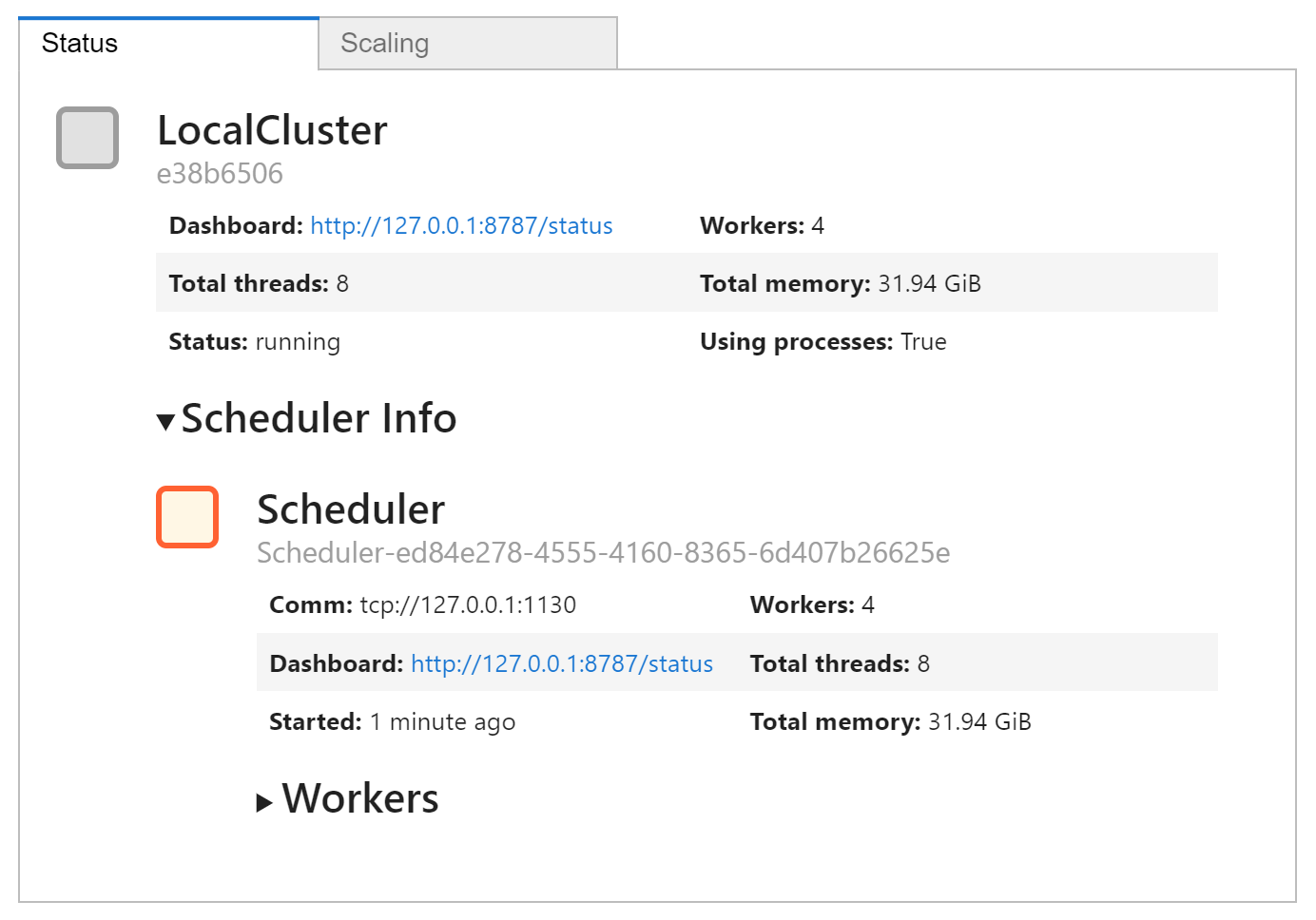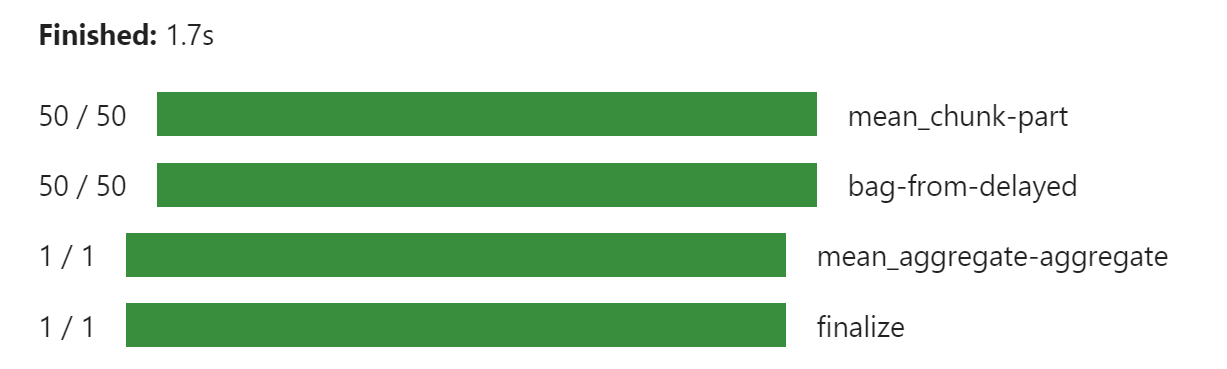## 分布式 futures

from dask.distributed import Client
c = Client(n_workers=4)
c.clusterdef inc(x):
return x + 1

fut = c.submit(inc, 1)
fut

Future: inc status: pending, type: None, key: inc-c5c7b9feb85235b658578a98acc14b70


fut

Future: inc status: finished, type: builtins.int, key: inc-c5c7b9feb85235b658578a98acc14b70


from dask.distributed import wait, progress
progress(fut)


wait(fut)


# 取回信息 - 如果 fut 没有准备好，这会阻塞
c.gather(fut)
# 只考虑一个 future 时的等效动作
# fut.result()

2


# 一些需要时间的琐碎工作
# 来自分布式章节

import time

def inc(x):
time.sleep(5)
return x + 1

def dec(x):
time.sleep(3)
return x - 1

time.sleep(7)
return x + y

x = delayed(inc)(1)
y = delayed(dec)(2)


fut = c.compute(total)
fut

Future: add status: pending, type: None, key: add-f86e2138-9bb3-4eaf-8015-840e0afd2c04

c.gather(fut) # 等待直到结果生成

3


### Client.submit()

submit 接受一个函数和参数，将它们推送到集群，返回一个 Future 表示要计算的结果。 该函数被传递给一个工作进程进行评估。 请注意，此单元格立即返回，而计算可能仍在集群上进行。

fut = c.submit(inc, 1)
fut

Future: inc status: pending, type: None, key: inc-993322fa7180f3b3b7cb4feb3c5ab69e


### 练习：改用 Client.submit 重建上述延迟计算

from dask import delayed
import time

def inc(x):
time.sleep(5)
return x + 1

def dec(x):
time.sleep(3)
return x - 1

time.sleep(7)
return x + y

x = c.submit(inc, 1)
y = c.submit(dec, 2)
total

Future: add status: pending, type: None, key: add-132b2dd01fa80047a9bde283fd14250a

c.gather(total)

3


futures API 提供了一种 work submission 风格，可以轻松模拟许多人可能熟悉的 map/reduce 范式 (参见 c.map())。 由 future 代表的中间结果可以传递给新任务，而不必从集群本地拉取，并且可以将新工作分配给尚未开始的先前工作的输出。

%run prep.py -d accounts

import dask.bag as db
import os
from pathlib import Path
import json

filename = Path("data", "accounts.*.json.gz")

f = c.compute(
js.filter(lambda record: record["name"] == "Alice")
.pluck("transactions")
.flatten()
.pluck("amount")
.mean()
)

from dask.distributed import progress
# 注意为了显示，进度条必须是单元格中的最后一行
progress(f)# 获取结果
c.gather(f)

1250.3844879997416

# 通过删除 futures 来释放结果
del f, fut, x, y, total


### Persist

%run prep.py -d random

import h5py
from pathlib import Path

f = h5py.File(Path("data", "random.hdf5"), mode="r")
dset = f["/x"]

x = da.from_array(dset, chunks=(1000000,))

%time x.sum().compute()
%time x.sum().compute()

Wall time: 35.4 s
Wall time: 2.66 s
999980540.0


# 将 x 从一组 delayed 处方更改为一组指向 RAM 中数据的 futures
# 在 UI 仪表板上查看此内容。
x = c.persist(x)

%time x.sum().compute()
%time x.sum().compute()

Wall time: 2.35 s
Wall time: 1.43 s
999980540.0


del x


## 异步计算

# a simple function with interesting minima
import time

def rosenbrock(point):
"""
计算 resenbrock 函数，并返回点和结果
"""
time.sleep(0.1)
score = (1 - point)**2 + 2 * (point - point**2)**2
return point, score


from bokeh.io import output_notebook, push_notebook
from bokeh.models.sources import ColumnDataSource
from bokeh.plotting import figure, show
import numpy as np

output_notebook()

# 设置绘图背景
N = 500
x = np.linspace(-5, 5, N)
y = np.linspace(-5, 5, N)
xx, yy = np.meshgrid(x, y)
d = (1 - xx)**2 + 2 * (yy - xx**2)**2
d = np.log(d)

p = figure(
x_range=(-5, 5),
y_range=(-5, 5)
)
p.image(
image=[d],
x=-5,
y=-5,
dw=10,
dh=10,
palette="Spectral11"
)


from dask.distributed import as_completed
from random import uniform

scale = 5  # 初始随机扰动尺度
best_point = (0, 0)  # 初始猜测
best_score = float("inf")  # 目前最好的得分
startx = [uniform(-scale, scale) for _ in range(10)]
starty = [uniform(-scale, scale) for _ in range(10)]

# 设置绘图
source = ColumnDataSource({
"x": startx,
"y": starty,
"c": ["grey"] * 10
})
t = show(p, notebook_handle=True)

# 初始化 10 个随机点
futures = [c.submit(rosenbrock, (x, y)) for x, y in zip(startx, starty)]
iterator = as_completed(futures)

for res in iterator:
# 选择一个完成的点，是否有提高？
point, score = res.result()
if score < best_score:
beat_score, best_point = score, point
print(score, point)

x, y = best_point
newx, newy = (x + uniform(-scale, scale), y + uniform(-scale, scale))

# 更新绘图
source.stream({
"x": [newx],
"y": [newy],
"c": ["grey"]
}, rollover=20)
push_notebook(document=t)

# 添加新点，动态，在集群中工作
new_point = c.submit(rosenbrock, (newx, newy))

# 缩小搜索范围并考虑停止
scale *= 0.99
if scale < 0.001:
break
point


## 调试

from dask import delayed

@delayed
def ratio(a, b):
return a // b

ina = [5, 25, 30]
inb = [5, 5, 6]
out = delayed(sum)([ratio(a, b) for (a, b) in zip(ina, inb)])
f = c.compute(out)
f

Future: sum status: pending, type: None, key: sum-05163712-648b-41db-bd34-19ffae849ab2


c.gather(f)

11


ina = [5, 25, 30]
inb = [5, 0, 6]
out = delayed(sum)([ratio(a, b) for (a, b) in zip(ina, inb)])
f = c.compute(out)
c.gather(f)

...
ZeroDivisionError: integer division or modulo by zero


import dask
# 这里不要使用 c.compute(out)
# 我们特别不想要分布式调度器
out.compute()

...
ZeroDivisionError: integer division or modulo by zero

# 取消注释以进入事后 (post-mortem) 调试器
# %debug


c.recreate_error_locally(f)

---------------------------------------------------------------------------
ZeroDivisionError                         Traceback (most recent call last)
<ipython-input-29-37e6c8a5bc9f> in <module>
----> 1 c.recreate_error_locally(f)

~\Anaconda3\envs\nwpc-data\lib\site-packages\distributed\recreate_exceptions.py in recreate_error_locally(self, future)
175             self.client.loop, self._recreate_error_locally, future
176         )
--> 177         func(*args, **kwargs)

<ipython-input-25-daf1cba59e73> in ratio(a, b)
3 @delayed
4 def ratio(a, b):
----> 5     return a // b
6
7 ina = [5, 25, 30]

ZeroDivisionError: integer division or modulo by zero

# 取消注释以进入事后 (post-mortem) 调试器
# %debug

> <ipython-input-2-daf1cba59e73>(5)ratio()
3 @delayed
4 def ratio(a, b):
----> 5     return a // b
6
7 ina = [5, 25, 30]

ipdb>  exit


[
(k, v.state) for k, v
if v.exception is not None
]

[('ratio-2cc06ec2-95b4-4eaa-a467-70081a00ec68', 'erred')]


## 结束

c.shutdown()